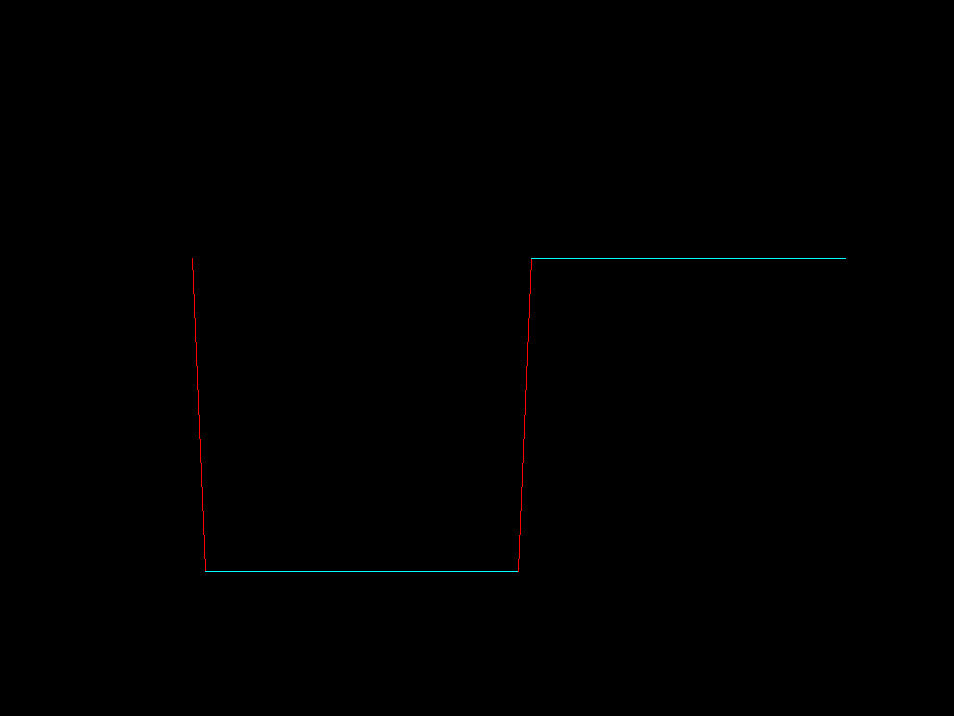# Dragon Curve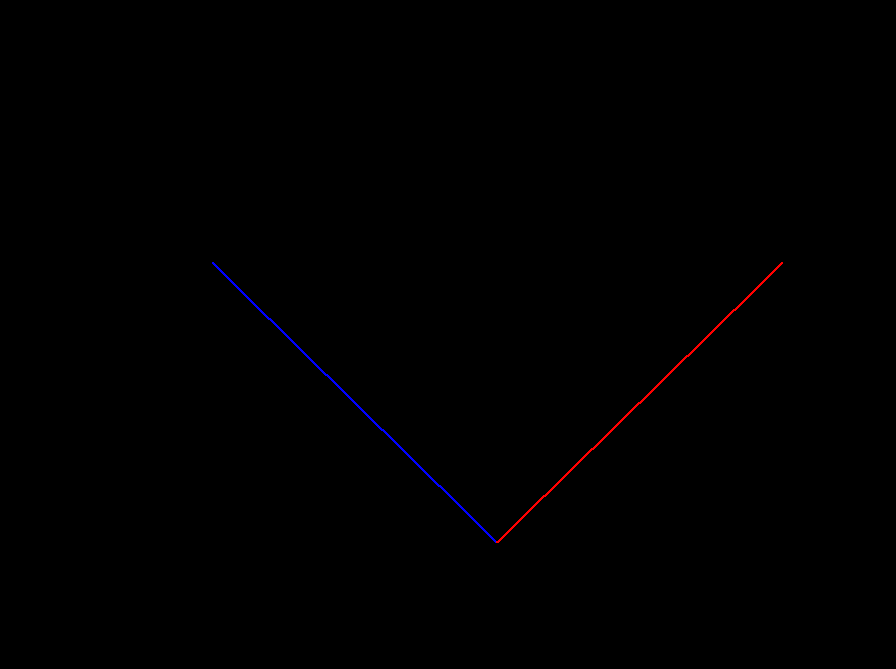Dragon curve construction The dragon is a fractal curve of Hausdorff dimension 2. One starts with one segment. In each iteration the number of segments is doubled by taking each segment as the diagonal of a square and replacing it by half the square, alternatingly to the left/right of the replaced segment. Here we increased the 90 degrees slightly so that you can better follow the curve. This also shows that the dragon lies in a family of fractal curves with Hausdorff dimension increasing from 1 to 2.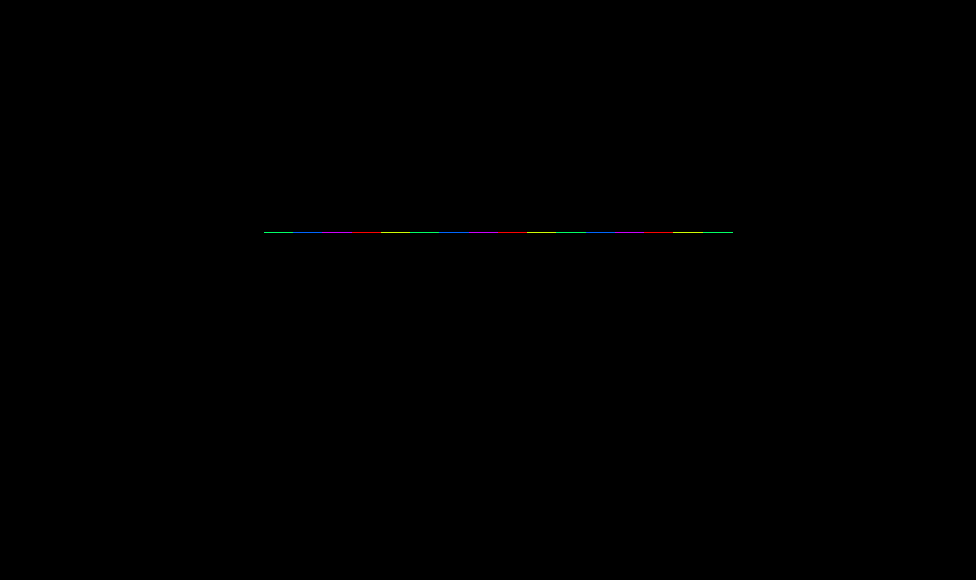In this animation the angle betwween the replacing edges decreases from 180 degrees to the 90 degrees of the dragon. We show the 4th iteration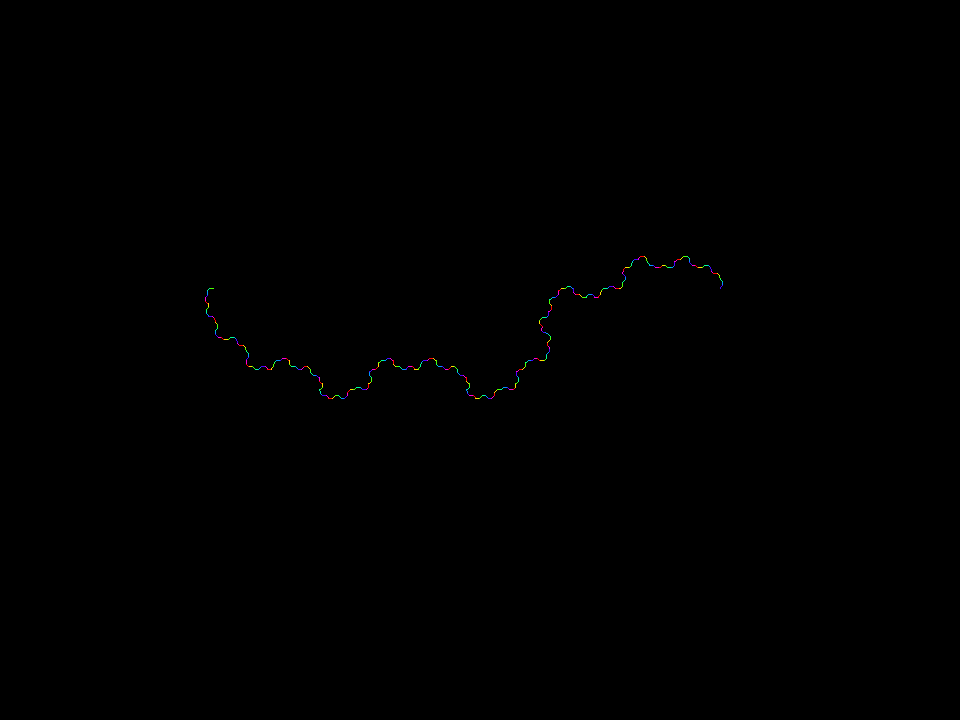Dragon angle morph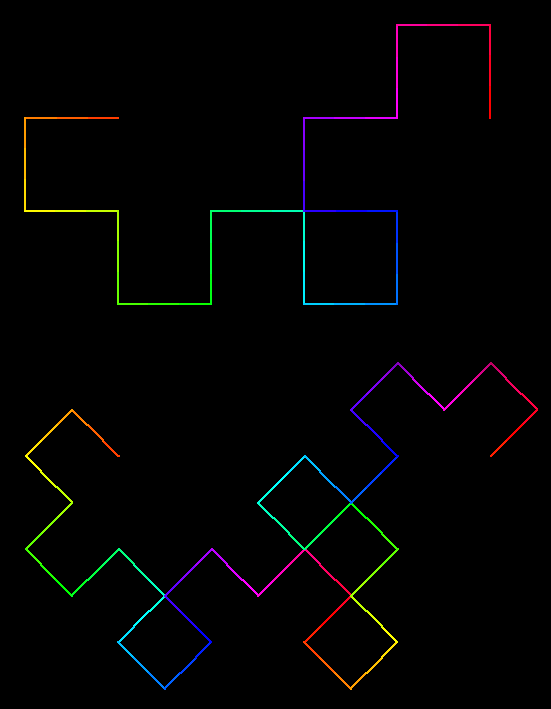The dragon curve has global construction. The image shows the 4th iteration (top) and the 5th iteration (bottom). The image of nth iteration is just 2 copies of the (n-1)th iteration, scaled by 1/sqrt(2) and rotated (clockwise) 45 degrees, and 135 degrees.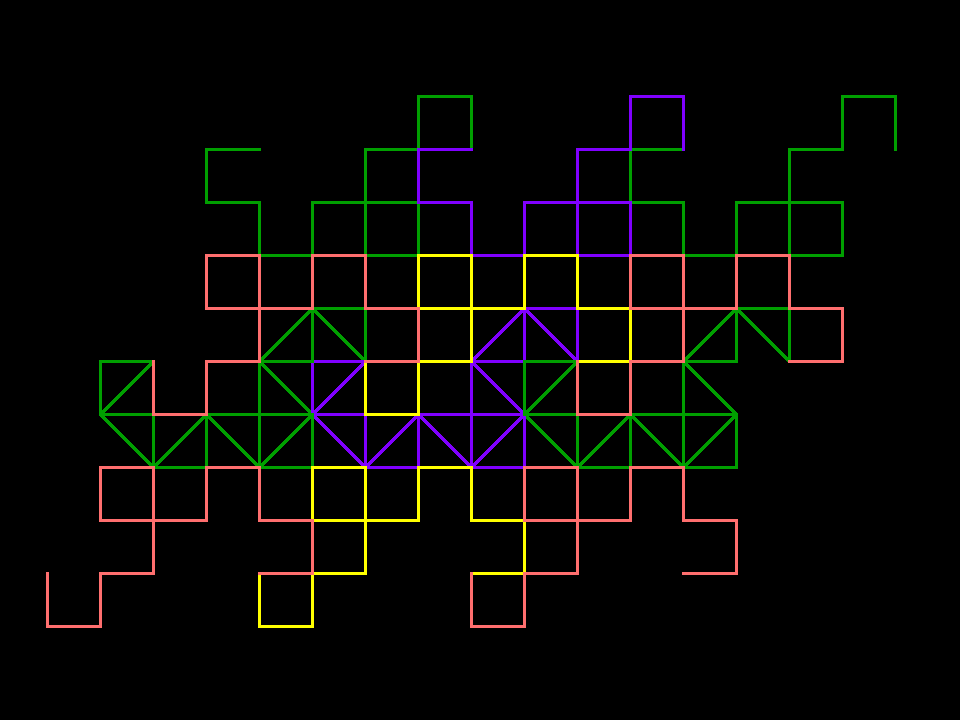In fact, the finite approximations of the dragon also “tile”. Strictly speaking, they cannot tile, because they are 1-dimensional non-closed polygons; but these fit together amzingly well and make it plausible that the limit dragon also tiles.